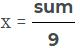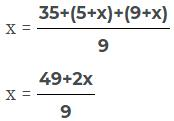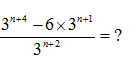Courses

# Average MCQ 2

## 20 Questions MCQ Test Quantitative Techniques for CLAT | Average MCQ 2

Description
This mock test of Average MCQ 2 for Quant helps you for every Quant entrance exam. This contains 20 Multiple Choice Questions for Quant Average MCQ 2 (mcq) to study with solutions a complete question bank. The solved questions answers in this Average MCQ 2 quiz give you a good mix of easy questions and tough questions. Quant students definitely take this Average MCQ 2 exercise for a better result in the exam. You can find other Average MCQ 2 extra questions, long questions & short questions for Quant on EduRev as well by searching above.
QUESTION: 1

### If the sum of five consecutive odd numbers is 255 then find the sum of largest and smallest number.

Solution:

SUm of 5 odd numbers = 255
Average = 255/5 = 51
47…..49…..51……53……55
Average = 51
Sum of largest and small number = 47+55 = 102

QUESTION: 2

### Nine students of a class contribute a certain sum. Seven of them give Rs. 5 each and the other two give Rs. 5 & 9 more then the average contribution of all the 9 students. The average contribution of the class of 9 students is.

Solution:

Average = sum/n
Average of 9 students = x
n = 9Seven of them give Rs. 5 = 7 x 5 = 35
35+(5+x)+(9+x)
∴ other two give Rs. 5 and Rs. 9 more than the average contribution9x = 49 + 2x
9x - 2x = 49
7x = 49
x = 7

QUESTION: 3

### Ranjan’s age is 1/3 of his father’s age.  After 10 years, his father’s age will be twice Raju’s age. If Raju’s eighth birthday was celebrated two years before, then Ranjan’s present age is.

Solution:
QUESTION: 4

There are total 600 students in a school. Average age of boys is 12 years and of girls is 11 years while average age of all students is 11 yrs. And 9 months. Find the number of girls in the school.

Solution:
QUESTION: 5

Ratio between present ages of A, B and C is 5:4:6. Total of the ages of A and C after 5 years will be 54 years. What will be the ratio of ages of B and C after 4 years?

Solution:
QUESTION: 6

Distance between two stations A & B is 778km. Hari Mohan covers the journey from A to B at 84km per hour and returns back to A with a uniform speed of 56km per hour. Find his average speed during the whole journey.

Solution:
QUESTION: 7

Sum of the ages of a father and son is 45 years. Five years, ago product of their ages was 4 times the father’s age at that time. Present ages of father and his son, respectively are:

Solution:
QUESTION: 8

Kamla got married 6 years ago. Today her age is 1(1/4) times her age at the time of marriage. Her son age is 1/10 times her age. Her son age is:

Solution:
QUESTION: 9

Maya’s age is 1/6 of her father’s age. Maya’s father’s age will be twice Vimal’s age after 10 years. If Vimal’s 8th birthday was celebrated two years ago, what is Maya’s present age?

Solution:
QUESTION: 10

Ratio of father’s age to his son’s age is 7:3. Product of their ages is 756. Ratio of their ages after 6 years will be

Solution:
QUESTION: 11

The ratio of Vipan’s age & Sonia’s age is 3:5 and the sum of their age is 80 years. The ratio of their ages after 10 years will be.

Solution:
QUESTION: 12

Average age of A&B is 24 years and average age of B, C and D is 22 years. The sum of ages of A, B, C and D is.

Solution:
QUESTION: 13

At present the age of father is five times that of the age of his son. Three years hence, the father age would be four times that of his son. Find the present age of his father and son.

Solution:
QUESTION: 14

A cricketer has completed 10 innings and his average is 21.5 runs. How many runs must he make in his next innings so as raise his average to 24?

Solution:
QUESTION: 15

The ratio of father’s age to the son’s age is 4:1 the product of their ages is 196. What will be the ratio of their ages after 5 years?

Solution:
QUESTION: 16

The ratio of the ages of father and the son at present is 6:1. After 5 years the ratio will become 7:2. What is present age of the son?

Solution:
QUESTION: 17

After 5 years, the age of father will be thrice the age of his son, whereas five years ago he was 7 times as old as his son was. What is the present age of son?

Solution:
QUESTION: 18Solution:
QUESTION: 19

5 years ago, the ratio of the ages of Neha and Rahul was 9:13. After five years, ratio their ages will be 11:15. What is the present age of Rahul?

Solution:
QUESTION: 20

The average age of a group of 10 students was 20. The average age increased by 2 years when two new students joined the group. What is the average age of the two new students who joined the group?

Solution:
The average age of a group of 10 students = 20 years
Sum of the ages of these 10 students = 10 * 20 = 200 years.
when 2 new students joined the average age is increased by = 2 years
Hence the average age becomes 22 years and total students become 12.
Therefore, the sum of the ages of the 12 students = 12 * 22 = 264 years
So, the difference between the sum of the ages of 12 students and 10 students = 264 - 200 = 64 years.
Sum of the ages of the 2 new students = 64 years.
Average age of the 2 new students = 64/2
= 32 years.